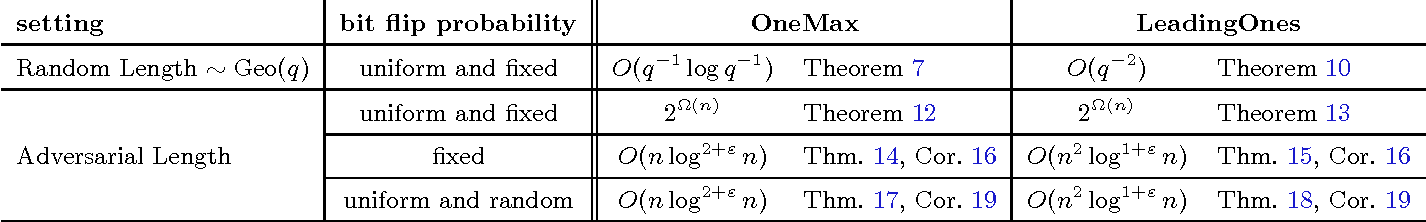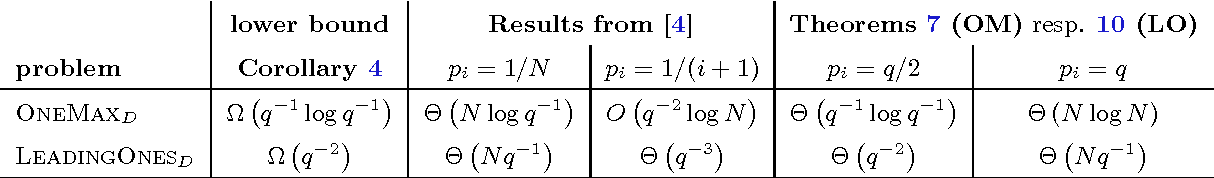Most research in the theory of evolutionary computation assumes that the problem at hand has a fixed problem size. This assumption does not always apply to real-world optimization challenges, where the length of an optimal solution may be unknown a priori. Following up on previous work of Cathabard, Lehre, and Yao [FOGA 2011] we analyze variants of the (1+1) evolutionary algorithm for problems with unknown solution length. For their setting, in which the solution length is sampled from a geometric distribution, we provide mutation rates that yield an expected optimization time that is of the same order as that of the (1+1) EA knowing the solution length. We then show that almost the same run times can be achieved even if \emph{no} a priori information on the solution length is available. Finally, we provide mutation rates suitable for settings in which neither the solution length nor the positions of the relevant bits are known. Again we obtain almost optimal run times for the \textsc{OneMax} and \textsc{LeadingOnes} test functions, thus solving an open problem from Cathabard et al.

* This is a preliminary version of a paper that is to appear at the Genetic and Evolutionary Computation Conference (GECCO 2015)Click To Get Code For Paper